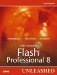# Nested Functions

 Nested functions can be a great tool if you want to run a repetitive set of scripts within a function but use the result differently in each function. Let's take a look at a couple of functionsthe first will square a user-defined number, and the second will combine two squared numbers by using the return value from the first function: ` function square(num:Number):Number{ return num*num; } //Now create the second function function combineSquares():Number{ var square1:Number = square(2); var square2:Number = square(3); return square1 + square2; } //Set the variable to the second function myNum = combineSquares(); trace(myNum); //output: 13 ` The preceding code uses the first function (square) and nests it within a second function (combineSquares) to return a value that uses the returned value of the square function. Now let's create a function that uses another function as a parameter. We'll use the same example as before, but this time we'll set the square function as a parameter: ` function square(num:Number):Number{ return num*num; } //Now create the second function function combineSquares(square1:Number, square2:Number):Number{ return square1 + square2; } //Set the variable to the second function myNum = combineSquares(square(2),square(3)); trace(myNum); //output: 13 ` As stated, you can nest functions within themselves for repetitive use if you want to use the result differently in each function. We have talked about creating and using functions in many ways; now let's see the scope of a function.Macromedia Flash Professional 8 Unleashed
ISBN: 0672327619
EAN: 2147483647
Year: 2005
Pages: 319